GeoA - chapter 1 Skills Maint 2
Angle A and Angle B are complementary. Angle A = 7x + 4, and angle B = 4x + 9. Find x. *
1 point
B is the midpoint of AC. Find the length of AC. You will need to find x first *
1 point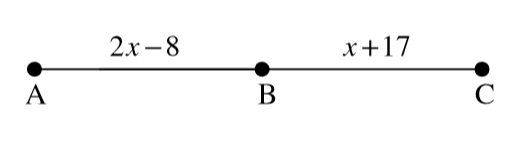Period *
Which option is the INCORRECT way to name this angle? *
1 point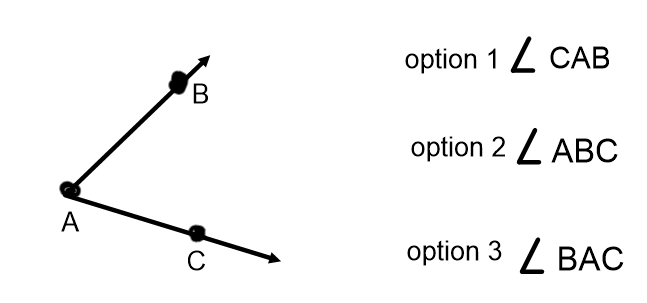Find x *
1 pointAngle A is 64 degrees. What is the measure of an angle that is supplementary to this? *
1 point
1 point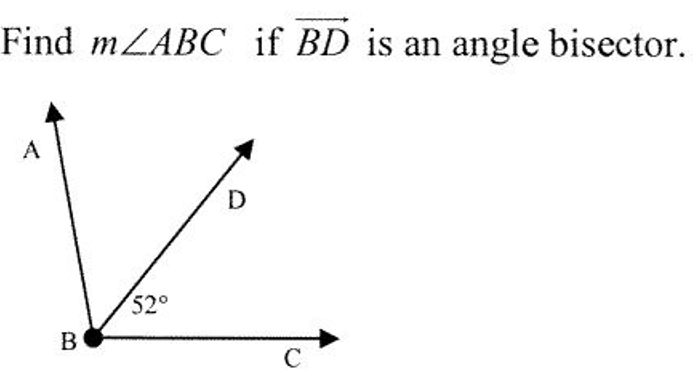Angle A and Angle B are supplementary. Angle A is a right angle. What is the measure of Angle B? *
1 point
Find c. *
1 point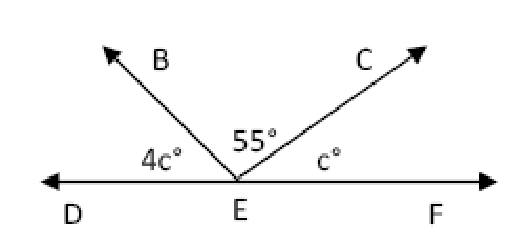What is the best description of a segment? *
1 point
Find x. *
1 point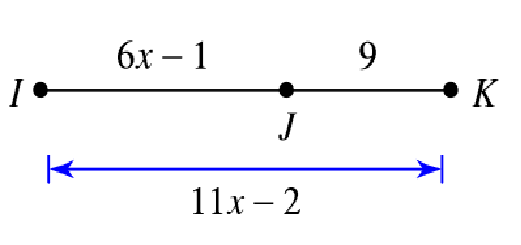Last Name *
Find the measure of angle x *
1 point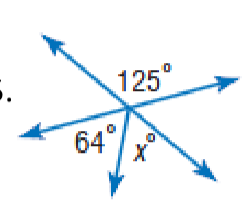Find measure of x. *
1 point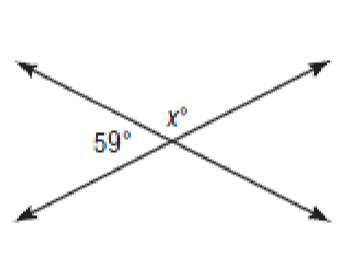Can two acute angles be supplementary? *
1 point
Find x *
1 point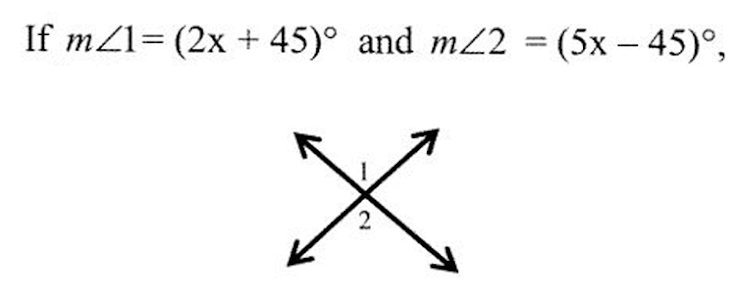Can two non adjacent angles be congruent? *
1 point